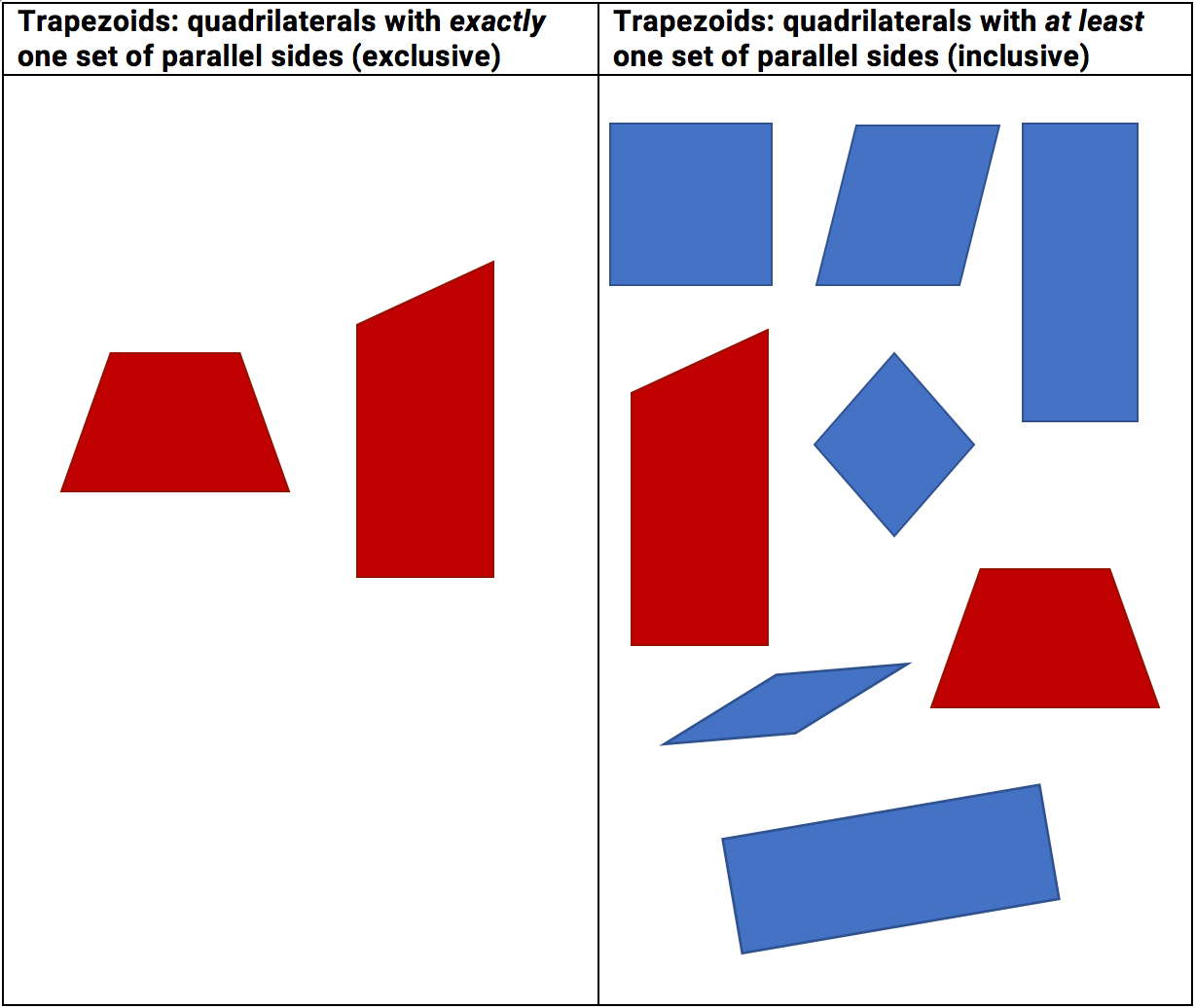Select Page

Question: Why did you decide to use the exclusive definition of a trapezoid?

Answer: As the question suggests, there is more than one definition of a trapezoid. Mathematicians define trapezoids in one of two ways:Using the inclusive definition, all parallelograms (which include rectangles, squares, and rhombuses) are trapezoids. Using the exclusive definition, they are not.

In determining which definition to use, we thought about a couple of things:

• Most elementary textbooks use the exclusive definition, and the Common Core State Standards do not specify which definition should be used. A sidebar in the geometry progressions states that most colleges/universities use the inclusive definition, but the mathematical goals at that level are different.
• Using the exclusive definition makes mathematical sense in the elementary grades. It supports one of the main mathematical goals of the two-dimensional geometry work in Investigations 3—getting students to think more deeply about the classification of 2-d shapes, and how changing an attribute or property can change how the shape is classified. Because this definition defines trapezoids as different from parallelograms, it complicates the hierarchy of 4-sided 2-d shapes in interesting ways—students need to identify not only parallel sides, but the number of parallel sides.

It often surprises people that there are two competing definitions. Isn’t mathematics supposed to be precise and have “one right answer”? This can be an interesting investigation to undertake with fourth or fifth grade students, who are often fascinated to learn of such mathematical disagreements. “What would happen if we change the rules, and use the definition of a trapezoid that says ‘at least one pair of parallel sides’? How would that change your categories and what shapes are in them”?

We are excited to have a space that offers us the opportunity to answer common questions from the field. Have questions you’d like to see answered? Email us.

Tag(s): definitions | geometry | Q&A |